The Princes

## The Princes

İngilizce’de fiyat sormak ya da fiyat söylemek için aşağıdaki yapılar kullanılır.
1. İlk olarak bilinmesi gereken para birimleridir.
’£ ‘(pound) İngiltere’nin büyük para birimidir. ’p’ (pence) İngiltere’nin küçük para birimidir.
100 p = 1 £
Write
1. 45 p + 43 p = 88 p
2. 65 p + 98 p = 1 £ and 63 p
3. 56 p + 78 p = ………
4. 26 p + 76 p = ………
5. 67 p + 9 p = ………
6. 89 p + 76 p = ………
7. 56 £ + 78 £ = ………
8. 78 p + 9 £ = ………
9. 67 £ + 90 p = ………
10. 67 p + 76 p = ………

3. 1£ 34 4. 1£ 2 5. 76 p 6. 1£ 65
7. 1£ 34 8. 9£ 78 9. 67£ 90 10. 1£ 43

2. Bir ürünün fiyatını sorarken;
– How much is/are ……………… ?
– How much do/does ……………. cost?

NOTE: ‘Pound’ olarak söylenen fiyatlar da ‘pence’ olarak bir miktar da varsa o miktar yanında ‘p’ ya da ‘pence’ olmadan yazılır.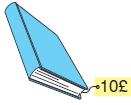A: How much is the book?
B: It is one pound and sixty seven pences.
A: How much does the book cost?
B: It costs one pound and sixty seven pences.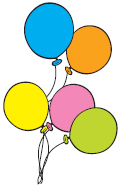A: How much are the balloons?
B: They are seventy eight pences.
A: How much do the balloons cost?
B: They cost seventy eight pences.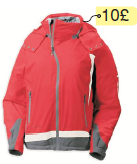A: How much is the coat?
B: It is ten pounds.
A: How much does the coat cost?
B: It costs ten pounds.

Activity Exercise

Change
Ex: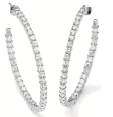How much are the earrings?
– How much do the earrings cost?
1.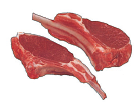How much is a kilo of meat?

2.
How much is a bunch of şowers?

3.
How much is a washing machine?

4.
How much are the lemons?

5.
How much is a Ferrari?

6.
How much are the planes?

7.
How much is a concert ticket?

8.
How much is that red dress?

9.
How much is this lighter?

1. How much does a kilo of meat cost?
2. How much does a bunch of flowers cost?
3. How much does a washing machine cost?
4. How much do the lemons cost?
5. How much does a Ferrari cost?
6. How much do the planes cost?
7. How much does a concert ticket cost?
8. How much does that red dress cost?
9. How much does this lighter cost?Sınavlara Hazırlık Arama Robotu
YGS & LYS TEOG KPSS TUS KPDS Ehliyet Sınavı PMYO JANA

Seçim esnek olup ilgili alanları seçiniz, Örneğin ehliyet sınavı için branş olarak matematik seçmeyiniz :)